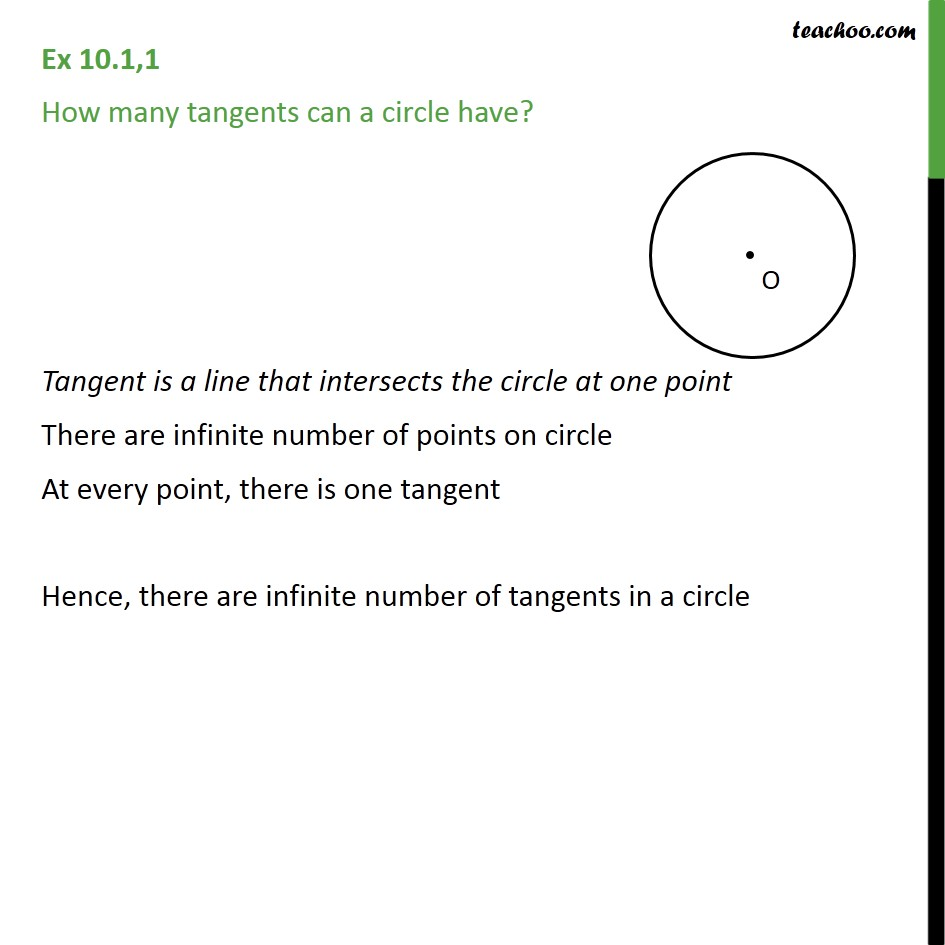Ex 10.1

Chapter 10 Class 10 Circles
Serial order wiseLearn in your speed, with individual attention - Teachoo Maths 1-on-1 Class

### Transcript

Ex 10.1,1 How many tangents can a circle have? Tangent is a line that intersects the circle at one point There are infinite number of points on circle At every point, there is one tangent Hence, there are infinite number of tangents in a circle